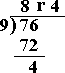Definition Of Remainder

Remainder is the amount left over after division when one divisor does not divide the dividend exactly.

Dividend = (Divisor × Quotient) + Remainder

Example of RemainderIn the above example, 2 is the divisor, 9 is the dividend, 4 is the quotient, and the left over, i.e. 1, is the remainder.

Solved Example on Remainder

Ques: Divide and write the answer with a remainder 76 ÷ 9.

Choices:

A. 7 r 4
B. 8 r 4
C. 4 r 8
D. 8 r 2